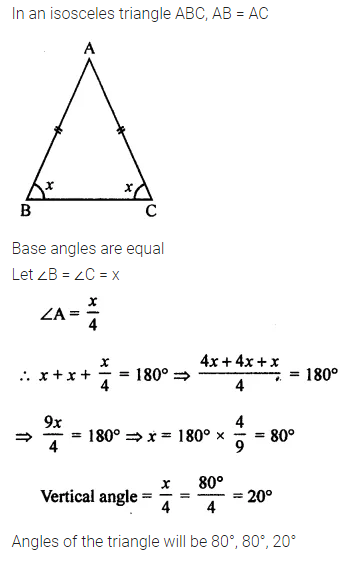ML Aggarwal Class 7 Solutions Chapter 11 Triangles and its Properties Ex 11.3 for ICSE Understanding Mathematics acts as the best resource during your learning and helps you score well in your exams.

## ML Aggarwal Class 7 Solutions for ICSE Maths Chapter 11 Triangles and its Properties Ex 11.3

Question 1.
Find the value of x in each of the following figures: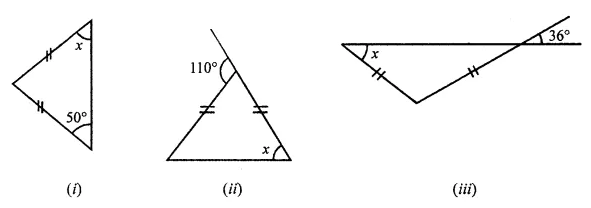Solution: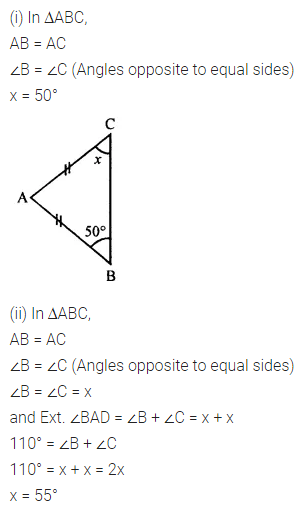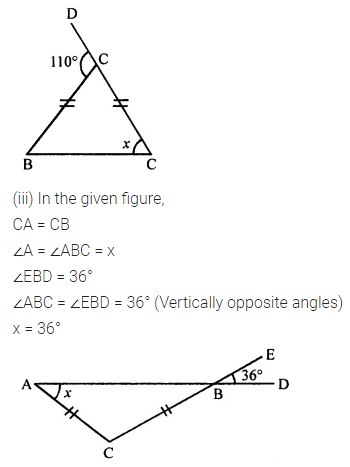Question 2.
Find the value of x in each of the following figures:Solution: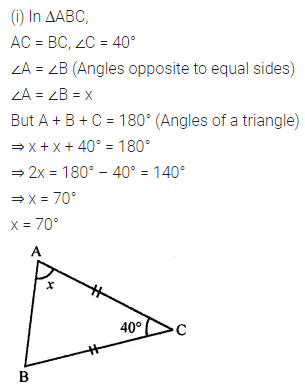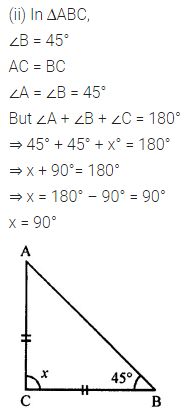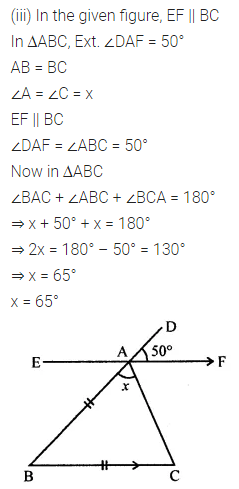Question 3.
Find the values of x and y in each of the following figures: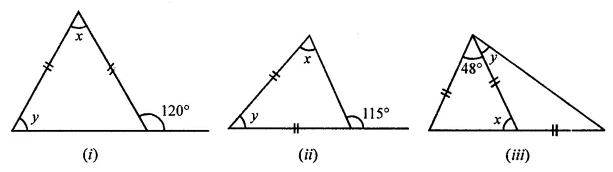Solution: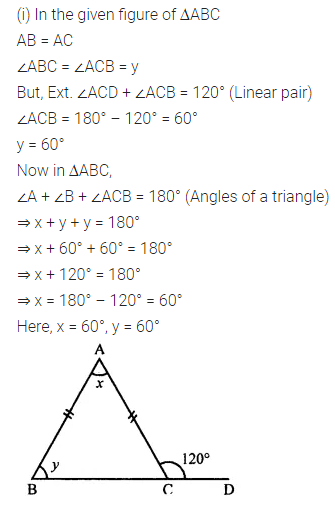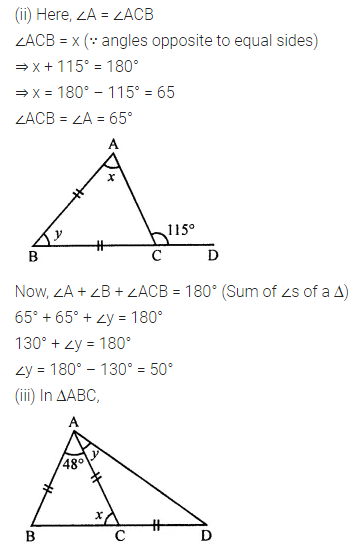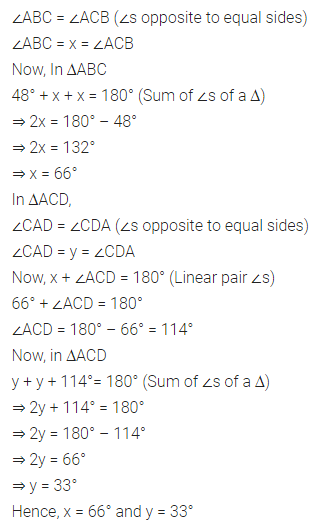Question 4.
Calculate the size of each lettered angle in the following figures: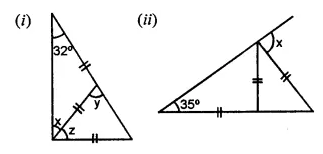Solution:Question 5.
If the angles of a triangle are in the ratio 1 : 2 : 1, find all the angles of the triangle. Classify the triangle in two different ways.
Solution:Question 6.
In an isosceles triangle, a base angle is four times its vertical angle. Find all the angles of the triangle.
Solution: